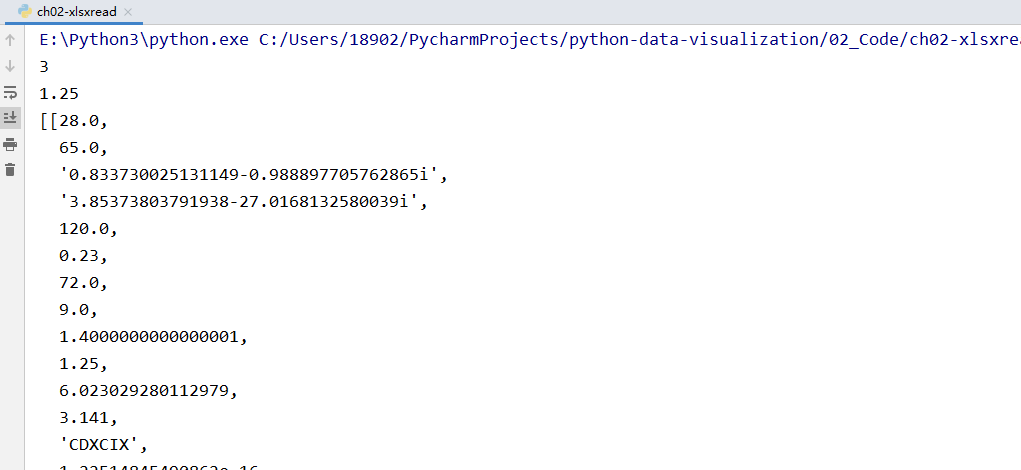## 2.3 如何从Microsoft Excel文件中导入数据

2021/2/6 22:23:03 文章标签: 测试文章如有侵权请发送至邮箱809451989@qq.com投诉后文章立即删除

## 如何从Microsoft Excel文件中导入数据

1、准备工作

2、操作步骤

• 打开文件的工作簿
• 根据名称找到工作表。根据行数（nrows）和列数（ncols）读取单元格的内容。
• 打印出了读取的数据集合

3、代码实现如下

``````import xlrd
from xlrd.xldate import XLDateAmbiguous

file='ch02-xlsxdata.xlsx'

wb=xlrd.open_workbook(filename=file)
ws=wb.sheet_by_name('Sheet1')

dataset=[]
for r in range(ws.nrows):
col=[]
for c in range(ws.ncols):
col.append(ws.cell(r,c).value)
if ws.cell_type(r,c)==xlrd.XL_CELL_DATE:
try:
print(ws.cell_type(r,c))
from datetime import datetime
data_value=xlrd.xldate_as_tuple(ws.cell(r,c).value,wb.datemode)
print(datetime(*data_value))
except XLDateAmbiguous as e:
print(e)
dataset.append(col)

from pprint import pprint
pprint(dataset)

``````

4、运行测试5、补充说明

暂无相关的数据...